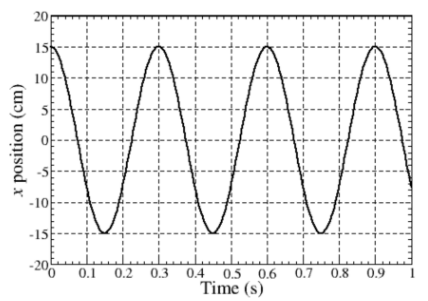# Problem: The graph below gives the position x vs. t for a spot on rim of a rotating wheel.Find:(a) The angular speed ω:(b) The speed of the spot v: (c) The magnitude of centripetal acceleration of the spot ac:(d) The magnitude of angular acceleration α: (e) The magnitude of tangential acceleration of the spot at:

###### FREE Expert Solution

(a)

The angular speed is expressed as:

$\overline{){\mathbf{\omega }}{\mathbf{=}}\frac{\mathbf{2}\mathbf{\pi }}{\mathbf{T}}}$,

88% (147 ratings)###### Problem Details

The graph below gives the position x vs. t for a spot on rim of a rotating wheel.Find:

(a) The angular speed ω:

(b) The speed of the spot v:

(c) The magnitude of centripetal acceleration of the spot ac:

(d) The magnitude of angular acceleration α:

(e) The magnitude of tangential acceleration of the spot at:

Frequently Asked Questions

What scientific concept do you need to know in order to solve this problem?

Our tutors have indicated that to solve this problem you will need to apply the Simple Harmonic Motion and Circular Motion concept. If you need more Simple Harmonic Motion and Circular Motion practice, you can also practice Simple Harmonic Motion and Circular Motion practice problems.